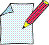Multiple choice test

Graph or not graphFor any of the following questions there is exactly one correct answer. You may click at any of the question marks to uncover whether the corresponding answer is the true or false. Take a sheet of paper whenever you feel this might help, in particular for the questions indicated by the symbol at the right. You may also this page and use it as worksheet. At the end of the document, you may activate an evaluation in terms of number of points achieved.

Hint to this test: The graph of a function  f  is the set of all points whose y-coordinate equals f (x), i.e. the number obtained when applying  f  to the x-coordinate. The x-axis is represented horizontally, the y-axis is represented vertically.

You have achieved    out of possible points.
Information on the evaluation system